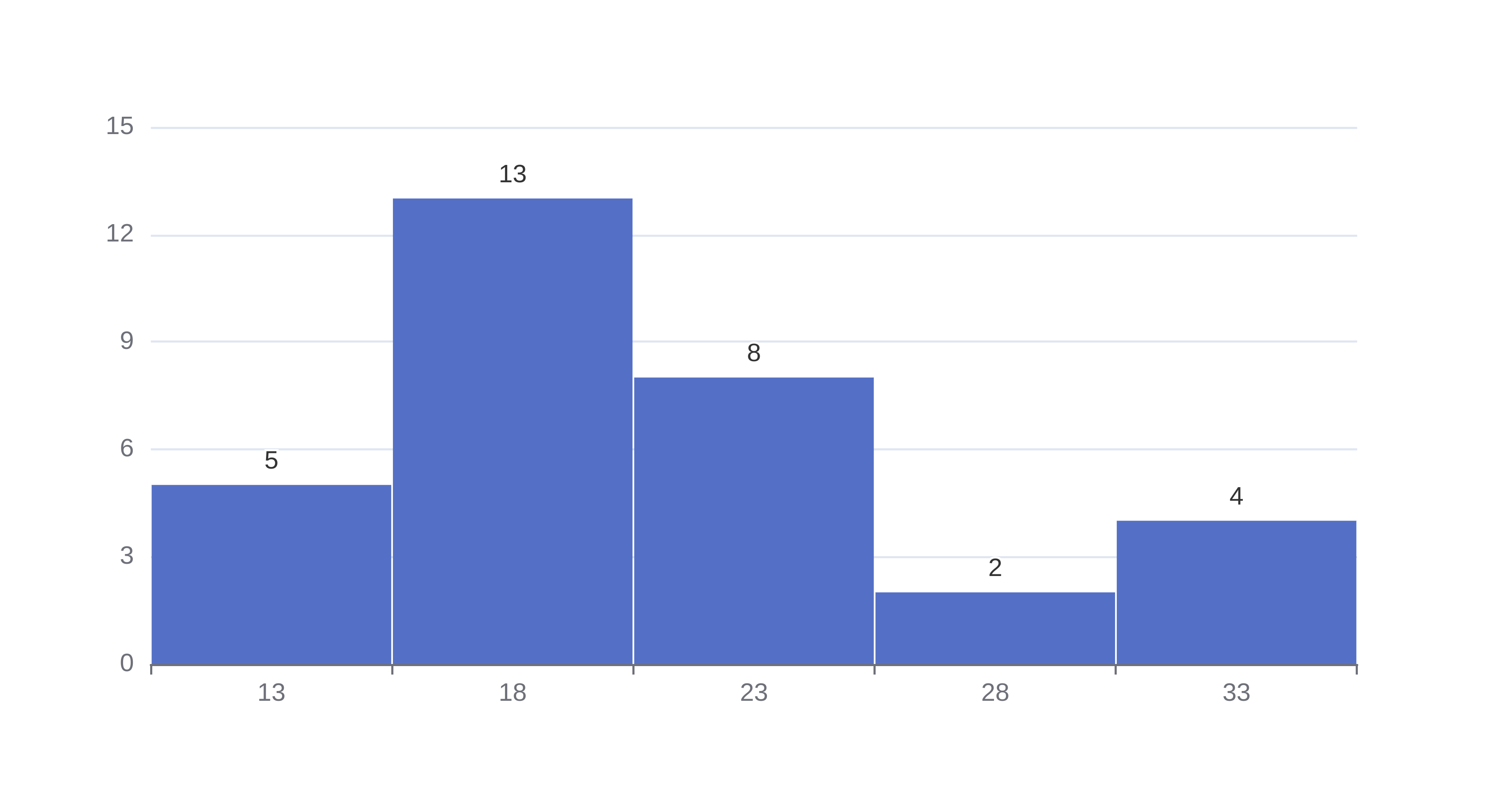# Histogram

Or input your data as csv

Sharing helps us build more free tools

Create a histogram with this free online histogram maker.

Histograms plot how often different numbers appear in a distribution using bars. They are often used to visualize distributions like the normal distribution.Histograms are one of my favorite numerical data visualization tools because they quickly show outliers and whether your data set is single or multi-modal.

A histogram is sometimes called a frequency distribution because it shows the frequencies that ranges of values from input continuous data appear. This is useful if you want to see what ranges of values appear most often in your data set.

This online histogram maker can be used to make a frequency distribution of the input continuous data.

## When To Use aHistogram

Histograms are really useful for visualizing distributions of data. For example, you might want to know how much rain your city gets. The mean gives you an average, but it does not tell you if there is a lot of rain on a few days per year, or if there is a little bit of rain every day. A histogram will show you whether there are a few days with a lot of rain or a lot of days with a little rain.

## How are Histograms Made From Numerical Data

Often in continuous data sets, each number might only occur once, but similar numbers might show up a lot. For example, we might have points for 1.01, 1.02, and 1.00. These numbers are all very close, but not exactly the same.

A histogram chart groups similar numbers together in bins on the x axis. Each bin has a width, e.g. 0.1. Most histograms have a fixed bin width. Some histograms, like exponential histograms, have a variable bin size that makes it easy to visualize distributions with a long tail.

Adding more bins to a histogram will show you the frequency distribution in more detail, but there might be bins without data. Removing bins will fill more bins up, but you won't be able to see as much detail about the data set.

The height of each bar on the y axis is controlled by how many numbers are within each bin. The bar width represents how big the range of values in the bin is. Since the height represents how many points are within the bin, histograms are sometimes called frequency distributions.

The highest bar on the y axis is the bin with the most values. Outliers appear in bins on the x axis that are short and furthest from the center of the histogram.